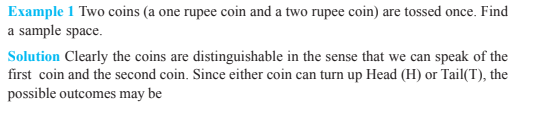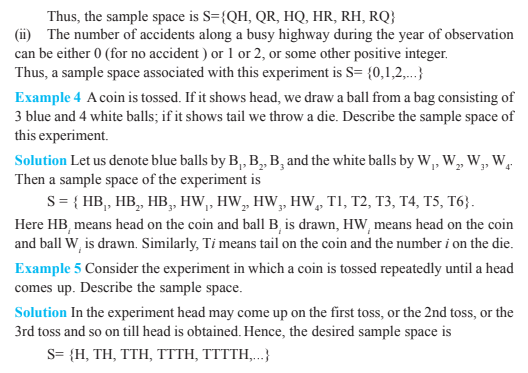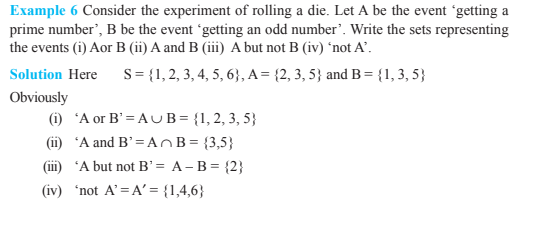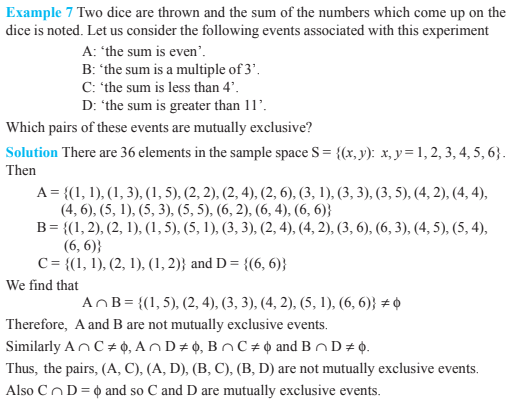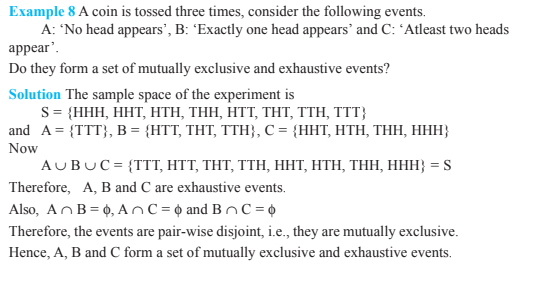# Probability Class 11 Notes - Chapter 16

Probability is an integral part of class 11 maths syllabus and has importance for not only the class 11 exams but also for different engineering exams like JEE. Here, a brief introduction to probability is given based on the class 11 maths syllabus which will help to learn the related concepts quickly and also to score in the exam.  Also, learn probability and statistics briefly here.

## Probability for Class 11: Key Concepts

• An experiment is said to be a random experiment if there are more than one possible outcomes and it’s impossible to predict the outcome in advance.
• All possible results of an experiment are called its outcome.
• Let us consider an experiment of rolling a die. All possible outcomes are 6, 5, 4, 3, 2, or 1. The set of all these outcomes {6, 5, 4, 3, 2, 1} is known as the sample space (denoted by ‘S’).
• Let us consider an experiment of tossing Two coins once. Since either coin can turn up Tail or Head, therefore, all the possible outcomes are Both coins – Head = HH, Both coins – Tail = TT, First coin – Head and Second coin – Tail = HT, First coin – Tail and second coin – Head = TH. Therefore, the sample space (S) can be represented as = {HT, TT, TH, HH,}.
• For any random experiment, Let S be the sample space. The probability P is a real-valued function whose domain is the power set of S and [0,1] is the range interval.
• For any event E, P(E)0
• P(S) = 1
• If E and F are mutually exclusive events, then P(E ∪ F) = P(E) + P(F)

### Events in Probability

The set of all possible outcomes is known as the Sample space. All elements of a sample space are known as Sample points. An event is a subset of the S (sample space). An empty set is also known as the Impossible event. The set A′ is known as the Complementary event.

• Event P or Q: The set P ∪ Q
• Event P and Q: The set P ∩ Q
• Event P and not Q: The set P – Q
• P and Q are mutually exclusive if P ∩ Q = φ
• Events P1, P2, . . . . . , Pn are exhaustive and mutually exclusive if P1 ∪ P2 ∪ . . . . . ∪ Pn = S and Ei ∩ Ej = φ V i ≠ j.

If P and Q are two events, then P(P or Q) = P(P) + P(Q) – P(P and Q) and P(P ∪ Q) = P(P) + P(Q) – P(P ∩ Q)

• If P and Q are mutually exclusive, then P(P or Q) = P(P) + P(Q)
• If M is an event, then P(not M) = 1 – P(M)

### Probability Class 11 Practice Questions